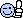<![CDATA[Math Is Fun Forum / Exercises]]> 2021-05-15T11:37:43Z FluxBB https://www.mathisfunforum.com/index.php <![CDATA[Compute the solution:]]> Hi GrasssyMilk,

Excellent!

938.

]]>
https://www.mathisfunforum.com/profile.php?id=682 2021-05-15T11:37:43Z https://www.mathisfunforum.com/viewtopic.php?id=21675&action=new
<![CDATA[Geometry For You]]> 59. Write the area A of a square as a function
of its perimeter P.

60. Write the area A of a circle as a function
of its circumference C.

61. Path of a Ball You throw a baseball to a child
25 feet away. The height y (in feet) of the baseball is
given by y = − (x^2)/10 x^2 + 3x + 6 where x is the horizontal
distance (in feet) from where you threw the ball.
Can the child catch the baseball while holding a baseball glove
at a height of 5 feet?

Enjoy.

]]>
https://www.mathisfunforum.com/profile.php?id=223890 2021-05-13T07:08:37Z https://www.mathisfunforum.com/viewtopic.php?id=26261&action=new
<![CDATA[Testing For Functions]]> In Exercises 9 and 10, which sets of ordered pairs represent
functions from A to B? Explain.

9. A = {0, 1, 2, 3} and B = {−2, −1, 0, 1, 2}

(a) {(0, 1), (1, −2), (2, 0), (3, 2)}
(b) {(0, −1), (2, 2), (1, −2), (3, 0), (1, 1)}
(c) {(0, 0), (1, 0), (2, 0), (3, 0)}
(d) {(0, 2), (3, 0), (1, 1)}

10. A = {a, b, c} and B = {0, 1, 2, 3}

(a) {(a, 1), (c, 2), (c, 3), (b, 3)}
(b) {(a, 1), (b, 2), (c, 3)}
(c) {(1, a), (0, a), (2, c), (3, b)}
(d) {(c, 0), (b, 0), (a, 3)}

NOTE: THIS IS EXTRA PRACTICE FOR MEMBERS. I AM NOT ASKING FOR HELP WITH 9 AND 10.

]]>
https://www.mathisfunforum.com/profile.php?id=223890 2021-05-13T07:04:49Z https://www.mathisfunforum.com/viewtopic.php?id=26260&action=new
<![CDATA[Find Distance Between Points]]> Not Dav wrote:
mathland wrote:

Find the distance between the points.

17. (−2, 6), (3, −6)

18. (8, 5), (0, 20)

19. (1, 4), (−5, −1)

20. (1, 3), (3, −2)

21. (1/2, 4/3) (2, −1)

22. (9.5, −2.6), (−3.9, 8.2)

___________________
would the formula be √ (x1 - x2)² + (y1 -y2)²    ? edit: oop the link already said so

I posted the problems as extra practice for members.

]]>
https://www.mathisfunforum.com/profile.php?id=223890 2021-05-13T07:00:40Z https://www.mathisfunforum.com/viewtopic.php?id=26124&action=new
in which (x, y) could be located.

9. x > 0 and y < 0

10. x < 0 and y < 0

11. x = −4 and y > 0

12. x < 0 and y = 7

13. x + y = 0, x ≠ 0, y ≠ 0

14. xy > 0

]]>
https://www.mathisfunforum.com/profile.php?id=223890 2021-04-22T08:11:41Z https://www.mathisfunforum.com/viewtopic.php?id=26122&action=new
<![CDATA[find area]]> Now knowing the radius, here are 2 methods for finding the area of the shaded region:

In the formulas...
r = the radius of the circumscribed circle
i = the radius of the respective inscribed circle
s = the sagitta of the respective circular segment

Method A (Area of circular segments minus area of inscribed circles)
1. Total area of the 3 circular segments = 481.49203......respective segment areas = r²ArcCos((r - s)/r) - (r - s)√(2rs - s²)
2. Total area of the 3 inscribed circles R, M & L = 175.92919......respective circle areas = πi²

481.49203
- 175.92919
------------
=======

Method B (Area of circumscribed circle minus areas of inscribed objects)
1. Area of circumscribed circle = 756.47691......πr²
2. Total area of the 3 inscribed circles R, M & L = 175.92919......respective circle areas = πi²
3. Area of triangle ABC, using Heron's Formula on the 3 circular segment chord lengths AB, AC & BC = 274.98488......respective chord lengths = 2√(2sr - s²)

756.47691
- 175.92919
- 274.98488
------------
=======

Edit: I first had Method B as my only method (same as the one in my post #5), but then discovered Method A.

]]>
https://www.mathisfunforum.com/profile.php?id=40741 2021-02-20T01:32:00Z https://www.mathisfunforum.com/viewtopic.php?id=26016&action=new
<![CDATA[puzzle by Xodus]]> I am not yet claiming that is the lowest 'n'.  Still working on it.
http://www.mathisfunforum.com/misc.php?action=rules

]]>
https://www.mathisfunforum.com/profile.php?id=222445 2020-08-06T09:11:57Z https://www.mathisfunforum.com/viewtopic.php?id=25773&action=new
<![CDATA[Statistics]]> Could you(i.e Zeeshan 01) take a look at some statistical concepts and their definitions? This is NOT a "we solve your problems, no strings attached~~" kind of deal, this is a "do your work and we'll help ya out." subforum..

]]>
https://www.mathisfunforum.com/profile.php?id=188456 2020-06-11T15:43:36Z https://www.mathisfunforum.com/viewtopic.php?id=25708&action=new
<![CDATA[Integration by Substitution]]> Zhylliolom wrote:

Hi,

I wasn't able to solve this using substitution, but here's a solution I came up with:

]]>
https://www.mathisfunforum.com/profile.php?id=118786 2020-05-17T00:07:35Z https://www.mathisfunforum.com/viewtopic.php?id=4208&action=new
<![CDATA[..find X]]> Ahhh, I see it now! Thanks, Bob!]]>
https://www.mathisfunforum.com/profile.php?id=118786 2020-05-16T19:27:38Z https://www.mathisfunforum.com/viewtopic.php?id=25644&action=new
<![CDATA[Measure Theory]]> zetafunc wrote:

#4

Hi,

]]>
https://www.mathisfunforum.com/profile.php?id=118786 2020-05-15T20:34:26Z https://www.mathisfunforum.com/viewtopic.php?id=23094&action=new
<![CDATA[log equation]]> Hi,

]]>
https://www.mathisfunforum.com/profile.php?id=118786 2020-05-15T19:47:27Z https://www.mathisfunforum.com/viewtopic.php?id=24702&action=new
<![CDATA[find area]]> hi Tony,

Eek! That's an evil looking question.  I'm assuming that the outer shape must be a quadrilateral otherwise the midpoint information is meaningless.  I am not assuming the shape is either a square or a rectangle.

Thanks for the puzzles.  Still working on the other one.

Bob

]]>
https://www.mathisfunforum.com/profile.php?id=67694 2020-04-30T14:10:28Z https://www.mathisfunforum.com/viewtopic.php?id=25643&action=new
<![CDATA[find AB]]> Hi Bob;

Thanks, that works perfectly well...and nice proof!The formula solves AB for a range of BMs (can be non-integer) without using the 3:4:5 triangle rule. LK is always 3.]]>
https://www.mathisfunforum.com/profile.php?id=40741 2020-04-23T15:51:26Z https://www.mathisfunforum.com/viewtopic.php?id=25581&action=new
<![CDATA[Find all pairs (x,y)]]> Thanks,

Bob

]]>
https://www.mathisfunforum.com/profile.php?id=67694 2020-03-30T09:08:54Z https://www.mathisfunforum.com/viewtopic.php?id=25567&action=new